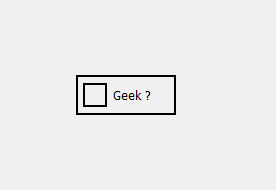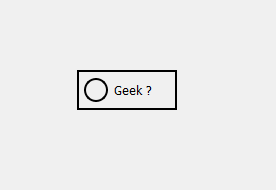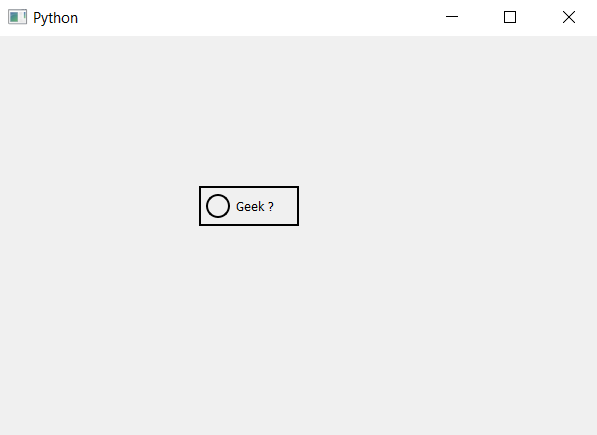# PyQt5 – Round indicator of CheckBox

• Last Updated : 22 Apr, 2020

In this article we will see how to create round indicator of check box. By default, check box have square indicator although with the help of style sheet we can change it make it round. Below is the representation of normal indicator vs the round indicator of check box.In order to do this we have to do the following :

1. Setting up the border to indicator as when we try to change the shape border will get vanish, so in order to deal with it we will provide custom border to it.
2. Set the size of indicator and it should be in square shape.
3. Set the border radius to sum of half of the length of indicator and the thickness of border i.e
`radius = width/2 + thickness`

Style sheet code –

```QCheckBox::indicator
{
border : 2px solid black;
width : 20px;
height : 20px;
}
```

Below is the implementation –

 `# importing libraries``from` `PyQt5.QtWidgets ``import` `*` `from` `PyQt5 ``import` `QtCore, QtGui``from` `PyQt5.QtGui ``import` `*` `from` `PyQt5.QtCore ``import` `*` `import` `sys`` ` ` ` `class` `Window(QMainWindow):`` ` `    ``def` `__init__(``self``):``        ``super``().__init__()`` ` `        ``# setting title``        ``self``.setWindowTitle(``"Python "``)`` ` `        ``# setting geometry``        ``self``.setGeometry(``100``, ``100``, ``600``, ``400``)`` ` `        ``# calling method``        ``self``.UiComponents()`` ` `        ``# showing all the widgets``        ``self``.show()`` ` `    ``# method for widgets``    ``def` `UiComponents(``self``):``        ``# creating the check-box``        ``checkbox ``=` `QCheckBox(``'Geek ?'``, ``self``)`` ` `        ``# setting tristate check box``        ``checkbox.setTristate(``True``)`` ` `        ``# setting geometry of check box``        ``checkbox.setGeometry(``200``, ``150``, ``100``, ``40``)`` ` `        ``# adding border to the check box and padding``        ``# adding border to indicator and setting size``        ``# setting border radius``        ``checkbox.setStyleSheet(``"QCheckBox"``                               ``"{"``                               ``"border : 2px solid clack;"``                               ``"padding : 5px;"``                               ``"}"``                               ``"QCheckBox::indicator"``                               ``"{"``                               ``"border : 2px solid black;"``                               ``"width : 20px;"``                               ``"height : 20px;"``                               ``"border-radius :12px;"``                               ``"}"``)`` ` ` ` `# create pyqt5 app``App ``=` `QApplication(sys.argv)`` ` `# create the instance of our Window``window ``=` `Window()`` ` `# start the app``sys.exit(App.``exec``())`

Output :My Personal Notes arrow_drop_up